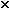# Fluid Statics/depth in a cylinder

## Homework Statement

Mercury is added to a cylindrical container to a depth d and then the rest of the cylinder is filled with water. If the cylinder is 0.8 m tall and the pressure at the bottom is 1.3 atmospheres, determine the depth of the mercury. (Assume the density of mercury to be 1.36104 kg/m3.)

## Homework Equations

Ptotal=Patmosphere+Pwater+Pmercury
P=density x g x height
1 atmosphere=1.013e5 Pa
density of water=1 x 103 kg/m3
pressure of atmosphere=1 atm

## The Attempt at a Solution

I first subtracted out the pressure of the atmosphere, so
.3 atm=Pwater + Pmercury
.8m=Hwater + Hmercury
Pmercury=1.36 x 104 x 9.8 x Hmercury
Pwater=1 x 103 x 9.8 x Hwater

I am having difficulty putting all 4 of these equations together. I feel like there is substitution needed, but which equations should I use?

kuruman
Homework Helper
Gold Member
Perhaps you should be more systematic, Starting with your equation
Ptotal=Patmosphere+Pwater+Pmercury
substitute
Pwaterwaterg hwater
Pmercurymercuryg hmercury
and solve for hmercury.

Chestermiller
Mentor

## Homework Statement

Mercury is added to a cylindrical container to a depth d and then the rest of the cylinder is filled with water. If the cylinder is 0.8 m tall and the pressure at the bottom is 1.3 atmospheres, determine the depth of the mercury. (Assume the density of mercury to be 1.36 View attachment 195815 104 kg/m3.)

## Homework Equations

Ptotal=Patmosphere+Pwater+Pmercury
P=density x g x height
1 atmosphere=1.013e5 Pa
density of water=1 x 103 kg/m3
pressure of atmosphere=1 atm

## The Attempt at a Solution

I first subtracted out the pressure of the atmosphere, so
.3 atm=Pwater + Pmercury
.8m=Hwater + Hmercury
Pmercury=1.36 x 104 x 9.8 x Hmercury
Pwater=1 x 103 x 9.8 x Hwater

I am having difficulty putting all 4 of these equations together. I feel like there is substitution needed, but which equations should I use?
You have 4 linear algebraic equations in 4 unknowns. Are you familiar with Gaussian elimination?

Perhaps you should be more systematic, Starting with your equation
Ptotal=Patmosphere+Pwater+Pmercury
substitute
Pwaterwaterg hwater
Pmercurymercuryg hmercury
and solve for hmercury.

Ok, if I do that
.3atm=(1000 x 9.8 x Hwater) + (1.36 x 104 x 9.8 x Hmercury)
I still need to find the height of water, right? Where would that come from?

You have 4 linear algebraic equations in 4 unknowns. Are you familiar with Gaussian elimination?
No, never heard of that. What is Gaussian elimination?

kuruman